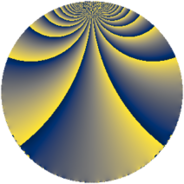# Properties

 Label 784.4.bpLevel $784$ Weight $4$ Character orbit 784.bp Rep. character $\chi_{784}(47,\cdot)$ Character field $\Q(\zeta_{42})$ Dimension $1008$ Sturm bound $448$

# Related objects

## Defining parameters

 Level: $$N$$ $$=$$ $$784 = 2^{4} \cdot 7^{2}$$ Weight: $$k$$ $$=$$ $$4$$ Character orbit: $$[\chi]$$ $$=$$ 784.bp (of order $$42$$ and degree $$12$$) Character conductor: $$\operatorname{cond}(\chi)$$ $$=$$ $$196$$ Character field: $$\Q(\zeta_{42})$$ Sturm bound: $$448$$

## Dimensions

The following table gives the dimensions of various subspaces of $$M_{4}(784, [\chi])$$.

Total New Old
Modular forms 4104 1008 3096
Cusp forms 3960 1008 2952
Eisenstein series 144 0 144

## Trace form

 $$1008q + 756q^{9} + O(q^{10})$$ $$1008q + 756q^{9} + 324q^{21} - 2352q^{25} - 420q^{29} + 108q^{33} + 2016q^{37} - 1980q^{45} - 480q^{49} + 588q^{53} + 168q^{57} + 4680q^{61} - 420q^{65} + 10920q^{69} + 324q^{73} - 816q^{77} - 588q^{81} - 1176q^{85} - 1908q^{89} - 756q^{93} + O(q^{100})$$

## Decomposition of $$S_{4}^{\mathrm{new}}(784, [\chi])$$ into newform subspaces

The newforms in this space have not yet been added to the LMFDB.

## Decomposition of $$S_{4}^{\mathrm{old}}(784, [\chi])$$ into lower level spaces

$$S_{4}^{\mathrm{old}}(784, [\chi]) \cong$$ $$S_{4}^{\mathrm{new}}(196, [\chi])$$$$^{\oplus 3}$$